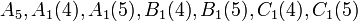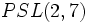# List of simple non-abelian groups of small order

Jump to: navigation, search

## Listing by order

The simple abelian groups are precisely the groups of prime order, and there is one such group for each prime number.

The first few simple non-abelian groups are listed below:

Group Order Number of conjugacy classes Families of simple non-abelian groups that it is a member of Shorthand notations
alternating group:A5 60 5 alternating group (parameter$n = 5$), projective special linear group ($PSL(2,4)$, also$PSL(2,5)$), projective symplectic group ($PSp(2,4), PSp(2,5)$), Chevalley group of type B ($B_1(4),B_1(5)$)$A_5, A_1(4),A_1(5),B_1(4),B_1(5),C_1(4),C_1(5)$
projective special linear group:PSL(3,2) 168 6 projective special linear group ($PSL(3,2)$, also$PSL(2,7)$), projective symplectic group ($PSp(2,7)$), Chevalley group of type B ($B_1(7)$)$A_2(2), A_1(7), B_1(7), C_1(7)$.
alternating group:A6 360 7 alternating group (parameter$n = 6$), projective special linear group ($PSL(2,9)$), projective symplectic group ($PSp(2,9)$), Chevalley group of type B ($B_1(9)$)$A_6, A_1(9), B_1(9), C_1(9)$. Also,$B_2(2)'$
projective special linear group:PSL(2,8) 504 9 projective special linear group ($PSL(2,8)$), Chevalley group of type B ($B_1(8)$), projective symplectic group ($PSp(2,8)$)$A_1(8), B_1(8), C_1(8)$
projective special linear group:PSL(2,11) 660 8 projective special linear group ($PSL(2,11)$),Chevalley group of type B ($B_1(11)$), projective symplectic group ($PSp(2,11)$)$A_1(11), B_1(11), C_1(11)$
projective special linear group:PSL(2,13) 1092 9 projective special linear group ($PSL(2,13)$),Chevalley group of type B ($B_1(13)$), projective symplectic group ($PSp(2,13)$)$A_1(13), B_1(13), C_1(13)$
projective special linear group:PSL(2,17) 2448 11 projective special linear group ($PSL(2,17)$),Chevalley group of type B ($B_1(17)$), projective symplectic group ($PSp(2,17)$)$A_1(17), B_1(17), C_1(17)$
alternating group:A7 2520 9 alternating group ($A_7$)$A_7$
projective special linear group:PSL(2,19) 3420 12 projective special linear group ($PSL(2,19)$), Chevalley group of type B ($B_1(19)$), projective symplectic group ($PSp(2,19)$)$A_1(19), B_1(19), C_1(19)$
projective special linear group:PSL(3,3) 5616 12 projective special linear group ($PSL(3,3)$)$A_2(3)$
projective special unitary group:PSU(3,3) 6048 14 projective special unitary group ($PSU(3,3)$)${}^2A_2(3^2)$
projective special linear group:PSL(2,23) 6072 14 projective special linear group ($PSL(2,23)$),Chevalley group of type B ($B_1(23)$), projective symplectic group ($PSp(2,23)$)$A_1(23), B_1(23), C_1(23)$
projective special linear group:PSL(2,25) 7800 15 projective special linear group ($PSL(2,25)$),Chevalley group of type B ($B_1(25)$), projective symplectic group ($PSp(2,25)$)$A_1(25), B_1(25), C_1(25)$
Mathieu group:M11 7920 10 sporadic simple group, among the Mathieu groups$M_{11}$